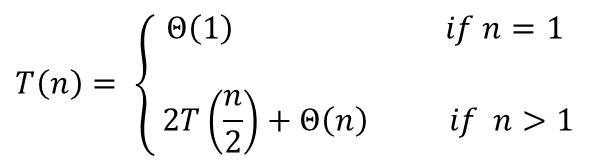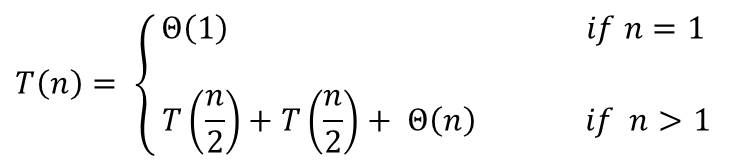# Recurrences in Divide and Conquer Algorithm

The divide and conquer class of algorithm solves a problem by recursively applying three basic steps at each stage of the recursion

Step 1: Divide the problem into two or more small subproblems

Step 2: Conquer these smaller problems by solving them using recursion. If the problem becomes small enough, just solve it.

Step 3: Combine the solutions derived from solving the subproblems into  a solution for the original problem

The Concept of Recurrence

A recurrence is a formula that gives a description of a function in terms of its in terms of its result on smaller inputs.  Recurrence provides a natural way of representing the running time of a divide and conquer algorithm.  An example of recurrence is the worse-case running time T(n) for the Merge-Sort algorithm when solved using recursion. This is given by the recurrence:The solution was found to be T(n) = Θ(n log n)

Recurrences can take on a number of forms. For instance a recursive algorithm may divide the subproblems into unequal sizes such as 2/3 to 1/3 splits. If the first two steps (divide and combine) steps take linear time, then the algorithm would result in  the recurrence

T(n) = T(2n/3) + T(n/3) + Θ(n)

Methods of Solving Recurrences

There are three methods of solving recurrences namely:

(i) The Substitution Method: In this method we made a guess of the bounds and then use mathematical induction to prove the guess

(ii) The Recursion-Tree Method: Here we convert the recurrences into a tree whose nodes represents the costs incurred are various stages of the recursion. Then techniques for bounding summations is used to solve the recurrence

(iii) The Master Method: In this method, bounds are provided for recurrences of the form:

T(n) = aT(n/b) + f(n)        –   (1.0)

where a ≥  1, b > 1 and f(n) is a given function.

This form of recurrence is an divide-and-conquer algorithm that creates a subproblems each being 1/b the size of the original problem and the divide and conquer steps take f(n) time to complete.

The Master Method can be used to determine the running time of the divide and conquer algorithm for the maximum subarray problem as well as for matrix multiplication.

More on Recurrences

Sometimes, recurrences could also be inequalities such as

T(n) ≤ 2T(n/2) + Θ(n)

And since this type of recurrence only states an upper bound on T(n), we would derive the solution using the O-notation instead of the Θ notation.

Another thing to know about recurrence is that there may be some difference between the actual subproblem size and the size used by the algorithm. Consider the merge sort algorithm for example for n elements. If n is odd, we conclude the subproblem sizes are n/2 and n/2. And these are not integers. Therefore, the worst-case for the running time for the Merge Sort is really:##### Check these Data Structure and Algorithms Tutorials#### kindsonthegenius

Kindson Munonye is currently completing his doctoral program in Software Engineering in Budapest University of Technology and Economics

View all posts by kindsonthegenius →